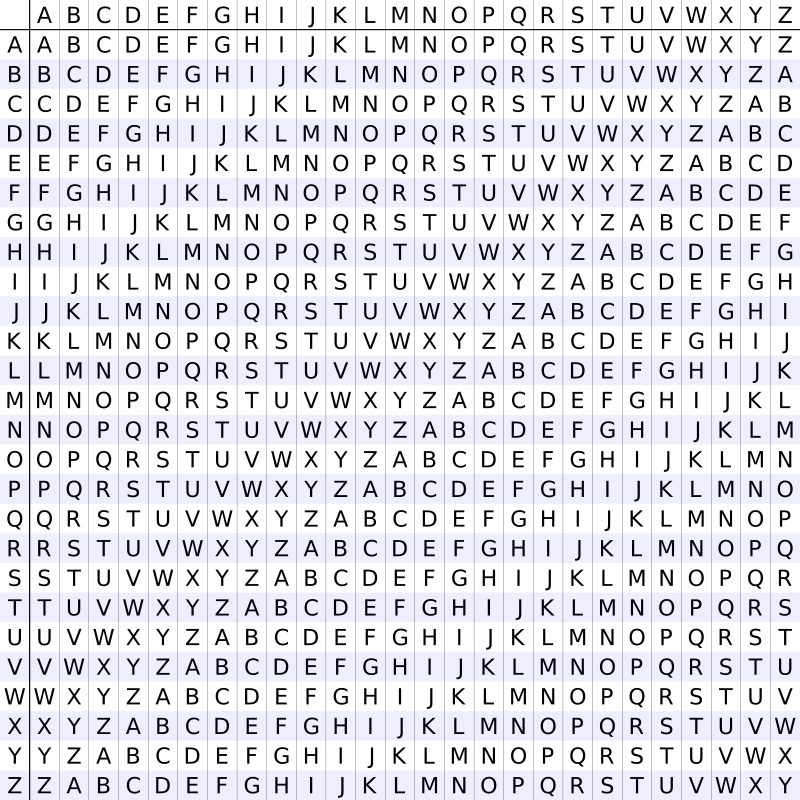# Vigenere Cipher in C and C++

In this tutorial you will learn about vigenere cipher in C and C++ for encryption and decryption.

Vigenere Cipher is kind of polyalphabetic substitution method. It is used for encryption of alphabetic text. For encryption and decryption Vigenere Cipher Table is used in which alphabets from A to Z are written in 26 rows.Also Read: Caesar Cipher in C and C++ [Encryption & Decryption]

Also Read: Hill Cipher in C and C++ (Encryption and Decryption)

### Vigenere Cipher Encryption

Message Text: THECRAZYPROGRAMMER

Key: HELLO

Here we have to obtain a new key by repeating the given key till its length become equal to original message length.

New Generated Key: HELLOHELLOHELLOHEL

For encryption take first letter of message and new key i.e. T and H. Take the alphabet in Vigenere Cipher Table where T row and H column coincides i.e. A.

Repeat the same process for all remaining alphabets in message text. Finally the encrypted message text is:

Encrypted Message: ALPNFHDJAFVKCLATIC

The algorithm can be expressed in algebraic form as given below. The cipher text can be generated by below equation.

E= (P+ Ki) mod 26

Here P is plain text and K is key.

### Vigenere Cipher Decryption

Encrypted Message: ALPNFHDJAFVKCLATIC

Key: HELLO

New Generated Key: HELLOHELLOHELLOHEL

Take first alphabet of encrypted message and generated key i.e. A and H. Analyze Vigenere Cipher Table, look for alphabet A in column H, the corresponding row will be the first alphabet of original message i.e. T.

Repeat the same process for all the alphabets in encrypted message.

Original Message: THECRAZYPROGRAMMER

Above process can be represented in algebraic form by following equation.

Pi = (E– Ki + 26) mod 26

We will use above algebraic equations in the program.

## Program for Vigenere Cipher in C

Output

Original Message: THECRAZYPROGRAMMER
Key: HELLO
New Generated Key: HELLOHELLOHELLOHEL
Encrypted Message: ALPNFHDJAFVKCLATIC
Decrypted Message: THECRAZYPROGRAMMER

## Program for Vigenere Cipher in C++

Comment below if you have queries or found anything incorrect in above tutorial for vigenere cipher in C and C++.

### 10 thoughts on “Vigenere Cipher in C and C++”

1.This is really a helpful article on Vigenere Cipher.

2.Can you explain the C code step by step specially the j and message[i] = “\0”;

And thanks

3.error : constant expression required
how to solve

4.constant expression required
how to solve

5.char newKey[msgLen], encryptedMsg[msgLen], decryptedMsg[msgLen];

you can’t create these arrays like this. c ++ wants a constant value for the sizes of these arrays. how did you compile this code?

1.You should use dynamic strings.
I mean:
int a = 0;
char* kelime = new char[a];

6.ERROR:constant expression required
how to solve the error
i need the code asap

7.Yeah the c++ does not work in the slightest. Encryption and Decryption both do not work.

8.In C++, array lengths must be declared as constant values at runtime. This is not a valid way of declaring arrays in in C++.
char newKey[msgLen], encryptedMsg[msgLen], decryptedMsg[msgLen];

If you’re going to publish your code online you should make sure it’s compilable first.

9.yeah it cant be run in VS code.
error: expression must have a constant value